Win up to 100% scholarship on Aakash BYJU'S JEE/NEET courses with ABNAT Win up to 100% scholarship on Aakash BYJU'S JEE/NEET courses with ABNAT

# 3D Geometry

## Introduction to Three Dimensional Geometry

3D geometry involves the Mathematics of shapes in 3D space and consists of 3 coordinates which are x-coordinate, y-coordinate and z-coordinate. In a 3d space, three parameters are required to find the exact location of a point. For JEE, three-dimensional geometry plays a major role, as a lot of questions are included from this topic. Here, the basic concepts of geometry involving 3-dimensional coordinates are covered, which will help students to understand different operations on a point in a 3d plane.

## Coordinate System in 3D Geometry

In 3 dimensional geometry, a coordinate system refers to the process of identifying the position or location of a point in the coordinate plane. To understand more about coordinate planes and systems, refer to coordinate geometry, which covers all the basic concepts, theorems, and formulas related to coordinate or analytic geometry.

### Rectangular Coordinate System

Three lines perpendicular to each other pass through a common point, it is called the origin, and the 3 lines are the axes: x-axis, y-axis, and z-axis, respectively. O is the observer, and with respect to its position, any other point is measured. The position or coordinates of any point in 3D space is measured by how much it has moved along the x, y and z-axis, respectively. So, if a point has a position (3, -4, 5), which means that it has moved 3 units along the positive x-axis, 4 units along the negative y-axis, and 5 units along the positive z-axis.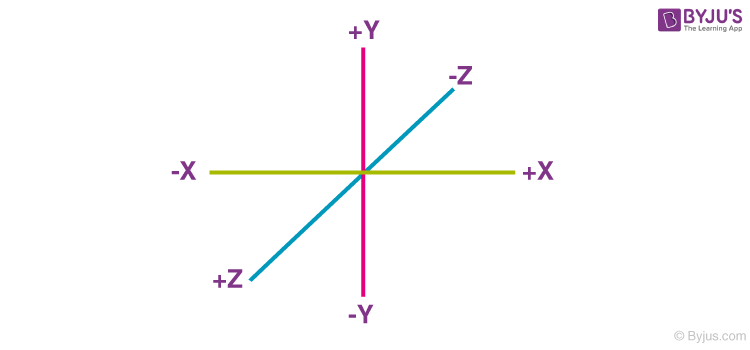Rectangular coordinate system – 3D Geometry

### Distance from the origin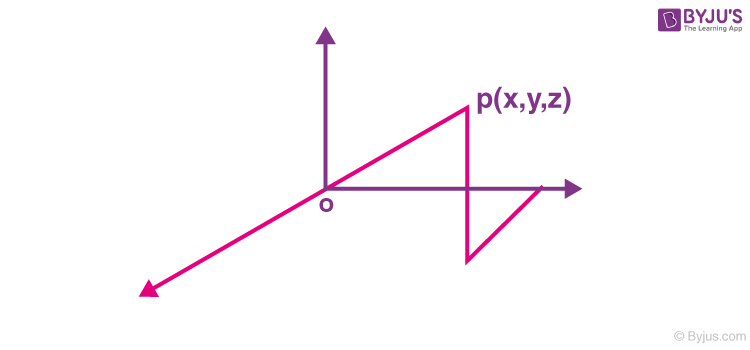Distance from the Origin in 3D Space – 3D Geometry

Distance from the origin. By using the Pythagoras theorem, the distance of P(x, y, z) from the origin (0, 0, 0) is:

$$\begin{array}{l}\sqrt{{{x}^{2}}+{{y}^{2}}+{{z}^{2}}}\end{array}$$

### Distance between 2 Points

Distance between 2 points P(x1, y1, z1) and Q(x2, y2, z2) is:

$$\begin{array}{l}PQ = \sqrt{{{\left( {{x}_{2}}-{{x}_{1}} \right)}^{2}}+{{\left( {{y}_{2}}-{{y}_{1}} \right)}^{2}}+{{\left( {{z}_{2}}-{{z}_{1}} \right)}^{2}}}\end{array}$$

### Division of a line joining 2 points

Let P(x1, y1, z1) and Q(x2, y2, z2) be 2 points. R derives the line segment PQ in ratio internally. Then, R has coordinates,

$$\begin{array}{l}\left( \frac{m{{x}_{2}}+n{{x}_{1}}}{m+n},\frac{m{{y}_{2}}+n{{y}_{1}}}{m+n}\frac{m{{z}_{2}}+n{{z}_{1}}}{m+n} \right)\end{array}$$

### Projection in 3D space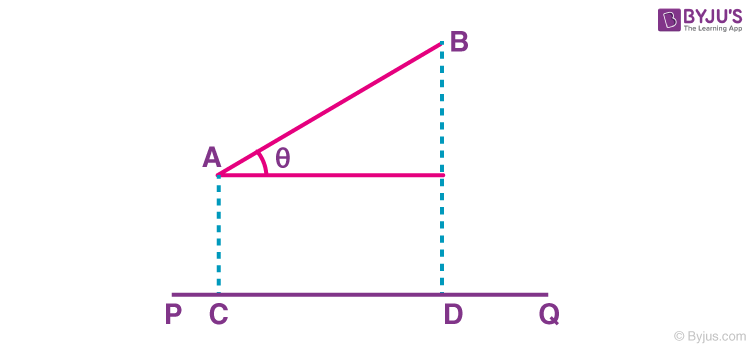Projection in 3D Space – 3D Geometry

Let AB be a line segment. It’s a projection on a line PQ, AB cos θ, where θ is the angle between AB and PQ or CD.

## Direction Cosines and Direction Ratios of a Line in the Cartesian Plane

Cosines of the angles a line makes with the positive x, y and z axis, respectively, are called direction cosines of that line.

So, if those angles are α, β and γ, then cos α, cos β, and cos γ are the direction cosines of the line. They are denoted by l, m, and n, respectively.

$$\begin{array}{l}{{l}^{2}}+{{m}^{2}}+{{n}^{2}}=1.\end{array}$$
(Proof will be given)

Any 3 numbers, a, b, and c, which are proportional to direction cosines, are called direction ratios.

Hence,

$$\begin{array}{l}\frac{l}{a}=\frac{m}{b}=\frac{n}{c}=\frac{\sqrt{{{l}^{2}}+{{m}^{2}}+{{n}^{2}}}}{\sqrt{{{a}^{2}}+{{b}^{2}}+{{c}^{2}}}}=\frac{1}{\sqrt{{{a}^{2}}+{{b}^{2}}+{{c}^{2}}}}\end{array}$$

$$\begin{array}{l}\therefore l=\frac{a}{\sqrt{\sum{{{a}^{2}}}}},m=\frac{b}{\sqrt{\sum{{{a}^{2}}}}},n=\frac{c}{\sqrt{\sum{{{a}^{2}}}}}\end{array}$$

### Direction cosine of line joining two given points

Let P(x1, y1, z1) and Q(x2, y2, z2) be 2 points. Then, direction cosines will be

$$\begin{array}{l}l=\frac{{{x}_{2}}-{{x}_{1}}}{\left| PQ \right|},m=\frac{{{y}_{2}}-{{y}_{1}}}{\left| PQ \right|},n=\frac{{{z}_{2}}-{{z}_{1}}}{\left| PQ \right|}\end{array}$$

### Projection of line segment joining 2 points on another line

Consider P(x1, y1, z1) and Q(x2, y2, z2).

Projection of PQ on a line whose direction cosines are l, m, n is

$$\begin{array}{l}l\left( {{x}_{2}}-{{x}_{1}} \right)+m\left( {{y}_{2}}-{{y}_{1}} \right)+n\left( {{z}_{2}}-{{z}_{1}} \right)\end{array}$$

### Angle between 2 lines in 3 dimensional space

2 lines having direction cosines (l1, m1, n1) and (l2, m2, n2). Then angle between them is

$$\begin{array}{l}\theta ={{\cos }^{-1}}\left( {{l}_{1}}{{l}_{2}}+{{m}_{1}}{{m}_{2}}+{{n}_{1}}{{n}_{2}} \right)\end{array}$$

### Projection of a plane area on 3 coordinate planes

$$\begin{array}{l}\text{Let}\ \bar{A}\ \text{be the vector area.}\end{array}$$
If its direction cosines are cos α, cos β, and cos γ. Then projections are
$$\begin{array}{l}{{A}_{1}}=A\cos \alpha ,{{A}_{2}}=A\cos \beta ,{{A}_{3}}=A\cos \gamma .\end{array}$$

$$\begin{array}{l}\therefore {{A}^{2}}=A_{1}^{2}+A_{2}^{2}+A_{3}^{2}\end{array}$$

### Area of a triangle

Using the projection formula, the area of a triangle,

$$\begin{array}{l}=\frac{1}{4}{{\left| \begin{matrix} {{x}_{1}} & {{y}_{1}} & 1 \\ {{x}_{2}} & {{y}_{2}} & 1 \\ {{x}_{3}} & {{y}_{3}} & 1 \\ \end{matrix} \right|}^{2}}+\,\,\frac{1}{4}{{\left| \begin{matrix} {{y}_{1}} & {{z}_{1}} & 1 \\ {{y}_{2}} & {{z}_{2}} & 1 \\ {{y}_{3}} & {{z}_{3}} & 1 \\ \end{matrix} \right|}^{2}}+\,\,\frac{1}{4}{{\left| \begin{matrix} {{x}_{1}} & {{z}_{1}} & 1 \\ {{x}_{2}} & {{z}_{2}} & 1 \\ {{x}_{3}} & {{z}_{3}} & 1 \\ \end{matrix} \right|}^{2}}\end{array}$$

Check out more details about the area of a triangle in coordinate geometry, its derivation and problem-solving strategies, etc.

## Concept of Plane in 3 Dimensional Geometry

A first-degree equation in x, y, z represents a plane in 3D

$$\begin{array}{l}ax+by+cz=0,{{z}^{2}}{{b}^{2}}+{{c}^{2}}\ne 0\end{array}$$
represents a plane.

### Normal form of a plane

Let P be the length of the normal from the origin to the plane and l, m, n be the direction cosines of that normal. Then, the equation of the plane is given by lx + my + nz = P.

### Intercept form

Let a plane cut lengths a, b, and c from the coordinate axis.

Then, the equation of the plane is:

$$\begin{array}{l}\frac{x}{a}+\frac{y}{b}+\frac{z}{c}=1\end{array}$$
.

### Planes passing through 3 given points

Plane passing through (x1, y1, z1), (x2, y2, z2) and (x3, y3, z3) is,

$$\begin{array}{l}\left| \begin{matrix} x & y & z & 1 \\ {{x}_{1}} & {{y}_{1}} & {{z}_{1}} & 1 \\ {{x}_{2}} & {{y}_{2}} & {{z}_{2}} & 1 \\ {{x}_{3}} & {{y}_{3}} & {{z}_{3}} & 1 \\ \end{matrix} \right|=0\end{array}$$

### Angle between 2 planes

a1x + b1y + c1z + d1 = 0 and a2x + b2y + c2z + d2 = 0 is given by

$$\begin{array}{l}\cos \theta =\frac{{{a}_{1}}{{a}_{2}}+{{b}_{1}}{{b}_{2}}+{{c}_{1}}{{c}_{2}}}{\sqrt{a_{1}^{2}+b_{1}^{2}+c_{1}^{2}}\sqrt{a_{2}^{2}+b_{2}^{2}+c_{2}^{2}}}\end{array}$$

### Two sides of a plane

Consider 2 points A(x1, y1, z1) and B(x2, y2, z2) lie on the same side or opposite sides of a plane ax + by + cz + d = 0, accordingly as

$$\begin{array}{l}a{{x}_{1}}+b{{y}_{1}}+c{{z}_{1}}+d\end{array}$$
and
$$\begin{array}{l}a{{x}_{2}}+b{{y}_{2}}+c{{z}_{2}}+d\end{array}$$
are of the same sign or opposite sign.

### Distance from a point to a plane

Distance of a point (x1, y1, z1) from a plane.

Distance of (x1, y1, z1) from ax + by + cz + d is

$$\begin{array}{l}\left| \frac{a{{x}_{1}}+b{{y}_{1}}+c{{z}_{1}}+d}{\sqrt{{{a}^{2}}+{{b}^{2}}+{{c}^{2}}}} \right|\end{array}$$

### Equation of the planes bisecting the angle between 2 planes

Let a1x + b1y + c1z + d1 = 0 and a2x + b2y + c2z + d2 = 0 be 2 planes. The equation of plane bisecting the angles between them is,

$$\begin{array}{l}\frac{{{a}_{1}}x+{{b}_{1}}y+{{c}_{1}}z+{{d}_{1}}}{\sqrt{a_{1}^{2}+b_{1}^{2}+c_{1}^{2}}}=\pm \frac{{{a}_{2}}x+{{b}_{2}}y+{{c}_{2}}z+{{d}_{2}}}{\sqrt{a_{2}^{2}+b_{2}^{2}+c_{2}^{2}}}\end{array}$$

### Position of origin

The origin lies in the acute or obtuse angle between a1x + b1y + c1z + d1 = 0 and a2x + b2y + c2z + d2 = 0 according as a1a2 + b1b2 + c1c2 < 0 or > 0  provided d1 and d2 are both positive.

### Two intersecting plane

If U = 0 and V = 0 be two planes, then the plane passing through the line of their intersection is U + λV = 0λ to be determined from the given condition.

## Straight lines in 3D

2 intersecting planes together represent a straight line.

### Equations of a straight line

• Equation of a straight line in symmetrical form

A straight line passing through (x1, y1, z1) and having direction cosines {l, m, n} is given by

$$\begin{array}{l}\frac{x-{{x}_{1}}}{l}=\frac{y-{{y}_{1}}}{m}=\frac{z-{{z}_{1}}}{n}\end{array}$$
• Two point form

Equation of a straight line passing through (x1, y1, z1) and (x2, y2, z2) is

$$\begin{array}{l}\frac{x-{{x}_{1}}}{{{x}_{2}}-{{x}_{1}}}=\frac{y-{{y}_{1}}}{{{y}_{2}}-{{y}_{1}}}=\frac{z-{{z}_{1}}}{{{z}_{2}}-{{z}_{1}}}\end{array}$$
• Two plane form to symmetrical form

Let 2 planes be a1x + b1y + c1z + d1 = 0 and a2x + b2y + c2z + d2 = 0 eliminate x to get a relation between y and z. Eliminate y to get the relation between y and z. Then, find in terms of x and find y in terms of z. Then, equate them.

### Intersection of a straight line and a plane

Let ax + by + cz + d = 0 is intersected by

$$\begin{array}{l}\frac{x-{{x}_{1}}}{l}=\frac{y-{{y}_{1}}}{m}=\frac{z-{{z}_{1}}}{n}.\end{array}$$

To find the intersection point, let

$$\begin{array}{l}\frac{x-{{x}_{1}}}{l}=\frac{y-{{y}_{1}}}{m}=\frac{z-{{z}_{1}}}{n}=t\end{array}$$

$$\begin{array}{l}\therefore x={{x}_{1}}+lt,y={{y}_{1}}+mt,z={{z}_{1}}+nt\end{array}$$
, put these in the equation of plane and solve for it.

### Plane through a given straight line

Let the line be

$$\begin{array}{l}\frac{x-{{x}_{1}}}{l}=\frac{y-{{y}_{1}}}{m}=\frac{z-{{z}_{1}}}{n}\end{array}$$
of the plane through this line be ax + by + cz + d = 0, then ax1 + by1 + cz1 + d = 0 and al + bm + cn = 0 and from other given conditions a, b, c are determined.

### Coplanarity of two lines in 3D geometry

Let 2 lines are

$$\begin{array}{l}\frac{x-{{x}_{1}}}{{{l}_{1}}}=\frac{y-{{y}_{1}}}{{{m}_{1}}}=\frac{z-{{z}_{1}}}{{{n}_{1}}}\,\,\And \,\,\frac{x-{{x}_{2}}}{{{l}_{2}}}=\frac{y-{{y}_{2}}}{{{m}_{2}}}=\frac{z-{{z}_{2}}}{{{n}_{2}}}\end{array}$$

Two lines are coplanar iff

$$\begin{array}{l}\left| \begin{matrix} {{x}_{2}}-{{x}_{1}} & {{y}_{2}}-{{y}_{1}} & {{z}_{2}}-{{z}_{1}} \\ {{l}_{1}} & {{m}_{1}} & {{n}_{1}} \\ {{l}_{2}} & {{m}_{2}} & {{n}_{2}} \\ \end{matrix} \right|=0\end{array}$$

### Distance of a point from a straight line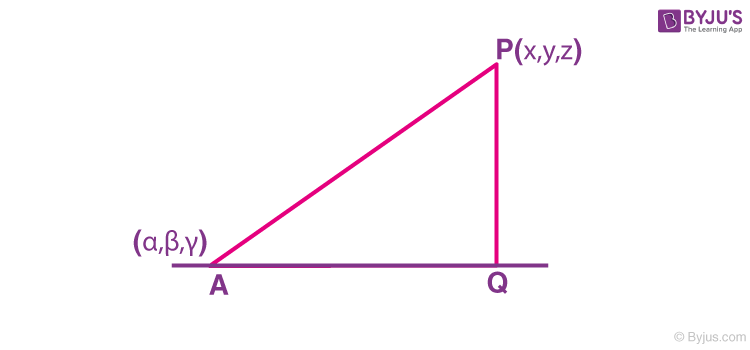Distance of a point from a straight line – 3D Geometry

Let the line be

$$\begin{array}{l}\frac{x-\alpha }{l}=\frac{y-\beta }{m}=\frac{z-\gamma }{n}\end{array}$$

AQ = projection of AP on the straight line

$$\begin{array}{l}=l\left( {{x}_{1}}\alpha \right)+m\left( {{y}_{1}}-\beta \right)+n\left( {{z}_{1}}-\gamma \right)\end{array}$$

$$\begin{array}{l}\therefore PQ=\sqrt{A{{P}^{2}}-A{{Q}^{2}}}\end{array}$$

### Shortest distance between two skew lines

Let the 2 skew lines be

$$\begin{array}{l}\frac{x-{{x}_{1}}}{{{l}_{1}}}=\frac{y-{{y}_{1}}}{{{m}_{1}}}=\frac{z-{{z}_{1}}}{{{n}_{1}}}\end{array}$$
and
$$\begin{array}{l}\frac{x-{{x}_{2}}}{{{l}_{2}}}=\frac{y-{{y}_{2}}}{{{m}_{2}}}=\frac{z-{{z}_{2}}}{{{n}_{2}}}\end{array}$$

The shortest distance is

$$\begin{array}{l}\frac{\left| \begin{matrix} {{x}_{2}}-{{x}_{1}} & {{y}_{2}}-{{y}_{1}} & {{z}_{2}}-{{z}_{1}} \\ {{l}_{1}} & {{m}_{1}} & {{n}_{1}} \\ {{l}_{2}} & {{m}_{2}} & {{n}_{2}} \\ \end{matrix} \right|}{\sqrt{\sum{{{\left( {{m}_{1}}{{n}_{2}}-{{m}_{2}}{{n}_{1}} \right)}^{2}}}}}\end{array}$$

The equation of the shortest distance is,

$$\begin{array}{l}\left| \begin{matrix} x-{{x}_{1}} & y-{{y}_{1}} & z-{{z}_{1}} \\ {{l}_{1}} & {{m}_{1}} & {{n}_{1}} \\ l & m & n \\ \end{matrix} \right|=0\end{array}$$

and

$$\begin{array}{l}\left| \begin{matrix} x-{{x}_{2}} & y-{{y}_{2}} & z-{{z}_{2}} \\ {{l}_{2}} & {{m}_{2}} & {{n}_{2}} \\ l & m & n \\ \end{matrix} \right|=0\end{array}$$

## Problems on 3D Geometry

Problem 1. If a variable plane forms a tetrahedron of constant volume 64 K3 with the coordinate planes, then the locus of the centroid of the tetrahedron is xyz = uK3. Find u.

Answer: Let the equation of the plane be

$$\begin{array}{l}\frac{x}{a}+\frac{y}{b}+\frac{z}{c}=1\end{array}$$

The centroid of the tetrahedron is (a/4, b/4, c/4).

Volume of the tetrahedron

$$\begin{array}{l}=\frac{abc}{6}=64{{K}^{3}}.\end{array}$$

So, letting

$$\begin{array}{l}\frac{a}{4}=x,\frac{b}{4}=y,\frac{c}{4}=z\end{array}$$

We have

$$\begin{array}{l}\frac{abc}{6}=\frac{{{4}^{3}}xyz}{6}=64{{K}^{3}}.\end{array}$$

∴ xyz = 6K3

On comparing, we have u = 6.

Problem 2. The ration in which the yz plane divides the line joining (2, 4, 5) and (3, 5, 7) is

Answer: Let the ratio be λ : 1.

x-coordinate  = 0

$$\begin{array}{l}\frac{3\lambda +2}{\lambda +1} = 0\end{array}$$

$$\begin{array}{l}\lambda =-\frac{2}{3}\end{array}$$

∴ The ratio is 2:3

Problem 3. A line makes angles α, β, γ, δ with the 4 diagonals of a cube, then

$$\begin{array}{l}\sum{{{\cos }^{2}}\alpha =?}\end{array}$$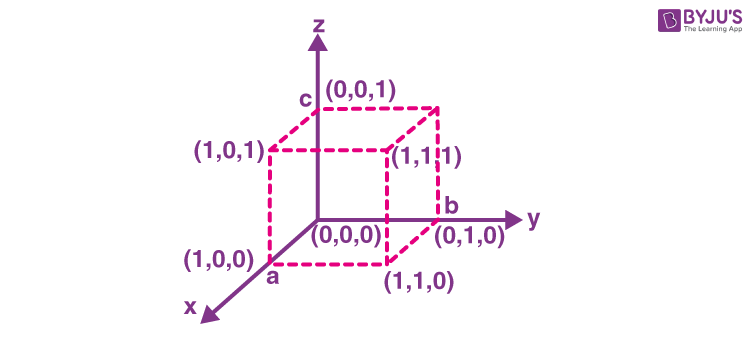Let the direction cosine of that line be (l, m, n).

Direction cosine of 1st diagonal (1/√3, 1/√3, 1/√3).

Direction cosine of 2nd diagonal (+1/√3, -1/√3, 1/√3).

Direction cosine of 3rd diagonal (1/√3, +1/√3, -1/√3) and

Direction cosine of 4th diagonal (1/√3, -1/√3, -1/√3)

$$\begin{array}{l}\therefore \cos \alpha =\frac{l}{\sqrt{3}}+\frac{m}{\sqrt{3}}+\frac{n}{\sqrt{3}}\end{array}$$

$$\begin{array}{l}\cos \beta =\frac{l-m+n}{\sqrt{3}},\cos \gamma =\frac{+l+m-n}{\sqrt{3}},\cos \delta =\frac{l-m-n}{\sqrt{3}}\end{array}$$

$$\begin{array}{l}\therefore \sum{{{\cos }^{2}}\alpha =\frac{4\left( {{l}^{2}}+{{m}^{2}}+{{n}^{2}} \right)}{3}}=\frac{4}{3}.\end{array}$$

Problem 4. The angle between the lines

$$\begin{array}{l}\frac{x-2}{3}=\frac{y+1}{-2}=\frac{z-2}{0}\ \text{and}\ \frac{x-1}{1}=\frac{2y+3}{3}=\frac{z+5}{2}\end{array}$$
is equal to

$$\begin{array}{l}\cos \theta =\frac{3\times1 – 2\times3/2+0\times2}{\sqrt{3^2+(-2)^2+0^2}\sqrt{1^2+(3/2)^2+2^2} }\end{array}$$

$$\begin{array}{l}\therefore \theta =\frac{\pi }{2}\end{array}$$

Problem 5. If lines

$$\begin{array}{l}\frac{x-1}{2}=\frac{y-2}{{{x}_{1}}}=\frac{z-3}{{{x}_{2}}}\ \text{and}\ \frac{x-2}{3}=\frac{y-3}{4}=\frac{z-4}{5}\end{array}$$
lies in the same plane then for the equation
$$\begin{array}{l}{{x}_{1}}{{t}^{2}}+\left( {{x}_{2}}+2 \right)t+a=0\end{array}$$
prove the sum of roots = -2.

$$\begin{array}{l}ax+by+cz+d=0\end{array}$$

$$\begin{array}{l}a+2b+3c+d=0\rightarrow{{}}\left( i \right)\end{array}$$

$$\begin{array}{l}2a+3b+4c+d=0\rightarrow{{}}\left( ii \right)\end{array}$$

$$\begin{array}{l}2a+{{x}_{1}}b+{{x}_{2}}c=0\rightarrow{{}}\left( iii \right)\end{array}$$

$$\begin{array}{l}3a+4b+5c=0\rightarrow{{}}\left( iv \right)\end{array}$$

$$\begin{array}{l}\text{Sum of the roots}=\frac{-\left( {{x}_{2}}+2 \right)}{{{x}_{1}}}\end{array}$$

From (i) and (ii),

a + b + c = 0

From (iii) and (iv),

a + b + c – d = 0

∴ d = 0

∴ a + 2b + 3c = 0

∴ b + 2c = 0

2a + 3b + 4c = 0

∴ b = 2c

3a + 4b + 5c = 0

2a – bc + 4c = 2c

2a = 2c

∴ a = c

Equation of the plane is ax + by + cz = 0 or cx + 2cy + cz = 0

or x – 2y = z = 0

$$\begin{array}{l}\therefore {{x}_{1}}=3{{x}_{2}}=4\end{array}$$

$$\begin{array}{l}\text{Sum of roots} =\frac{-\left( {{x}_{2}}+2 \right)}{{{x}_{1}}}\end{array}$$

= -6/3

= -2

Problem 6. The line x/K = y/2 = z/-12 makes an isosceles triangle with the planes 2x + y + 3z – 1 = 0 and x + 2y – 3z – 1 = 0 then K = ?

Answer: Equation of the bisector planes are

$$\begin{array}{l}\frac{2x+y+3z-1}{\sqrt{14}}=\pm \frac{x+2y-3z-1}{\sqrt{14}}\end{array}$$

i.e., 2x + y + 3z – 1 = x + 2y – 3z – 1

or x – y + 6z = 0…..(i)

and 2x + y + 3z – 1 = -x – 2y + 3z + 1

i.e., 3x + 3y – 2 = 0 …..(ii)

So, the given line must be parallel to (i) or (ii).

The coefficient of x and y of the required straight line equation is -1 and 1.
Therefore, the value of K is -2.

Problem 7. The direction cosines of normal to the plane containing lines x = y = z and x – 1 = y – 1 = (z – 1)/d are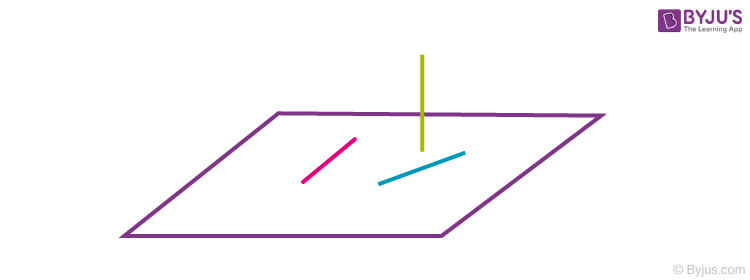Problems in 3D Geometry

Let directions cosines be l,m,n.

∴ l + m + n = 0 and l + m + dn = 0

i.e. n=0

∴ l = -m from l2 + m2 + n2 = 1

We have 2l2 = 1

$$\begin{array}{l}\therefore l=\pm \frac{1}{\sqrt{2}}\end{array}$$

∴ (l, m, n) is

$$\begin{array}{l}\left( \frac{1}{\sqrt{2}},-\frac{1}{\sqrt{2}},0 \right)\end{array}$$
or
$$\begin{array}{l}\left( -\frac{1}{\sqrt{2}},\frac{1}{\sqrt{2}},0 \right).\end{array}$$

Problem 8. If the line x = y = z intersect the line x sin A + y sin B + z sin C = 2d2, x sin 2A + y sin 2B + z sin 2C = d2, where (A + B + C = π), then

$$\begin{array}{l}\sin \frac{A}{2}.\sin \frac{B}{2}.\sin \frac{C}{2}=?\end{array}$$

Answer: Let the point of intersection be (t, t, t).

$$\begin{array}{l}\therefore \left( \sin A+\sin B+\sin C \right)t=2{{d}^{2}}\end{array}$$

$$\begin{array}{l}\left( \sin 2A+\sin 2B+\sin 2C \right)t={{d}^{2}}\end{array}$$

$$\begin{array}{l}\sin 2A+\sin 2B-\sin \left( 2A+2B \right)=\frac{{{d}^{2}}}{t}.\end{array}$$

$$\begin{array}{l}\Rightarrow 2.\sin \left( A+B \right).\cos \left( A-B \right)-2\sin \left( A+B \right).\cos \left( A+B \right)=\frac{{{d}^{2}}}{t}\end{array}$$

$$\begin{array}{l}\Rightarrow 4\sin C\,\,\sin A.\,\,\sin B=\frac{{{d}^{2}}}{t.}\rightarrow{{}}\left( i \right)\end{array}$$

Again,

$$\begin{array}{l}\sin A+\sin B+\sin C\end{array}$$

$$\begin{array}{l}=2.\sin \frac{A+B}{2}.\cos \frac{A-B}{2}+2\sin \frac{C}{2}.\cos \frac{C}{2}\end{array}$$

$$\begin{array}{l}=2\cos \frac{C}{2}.\cos \frac{A-B}{2}+2\sin \frac{C}{2}.\cos \frac{C}{2}\end{array}$$

$$\begin{array}{l}=2\cos \frac{C}{2}\left( \cos \frac{A-B}{2}+\cos \frac{A+B}{2} \right)\end{array}$$

$$\begin{array}{l}=2\cos \frac{C}{2}.2cos\frac{A}{2}.\cos \frac{B}{2}=\frac{2{{d}^{2}}}{t}\rightarrow{{}}\left( ii \right)\end{array}$$

dividing (i) by (ii),

$$\begin{array}{l}\sin \frac{A}{2}.\sin \frac{B}{2}.\sin \frac{C}{2}=\frac{1}{16}.\end{array}$$

Problem 9. Equation of the sphere having a centre at (3, 6, -4) and touching the plane

$$\begin{array}{l}\bar{r}.\left( 2\hat{i}-2\hat{j}-\hat{k} \right)=10\ \text{is}\ {{\left( x-3 \right)}^{2}}+{{\left( y-6 \right)}^{2}}+{{\left( z+4 \right)}^{2}}={{K}^{2}},\end{array}$$
then k =

Answer: Equation of the plane is 2x – 2y – z – 10 = 0

Distance from (3, 6, -4) to it is

$$\begin{array}{l}\left| \frac{6-12+4-10}{\sqrt{9}} \right|=\left| \frac{12}{3} \right|=4\end{array}$$

∴ k = 4.

### 3D Geometry – Complete JEE Questions### Vector Algebra and 3D Geometry – Important Topics### Vector Algebra and 3D Geometry – Important Questions### Vector Algebra and 3D Geometry – Important Topics Part 2### Vector Algebra and 3D Geometry – Important Questions Part 2### 3D Geometry – Top 10 Most Important and Expected JEE Questions### 3-D Geometry – JEE Advanced ProblemsQ1

### What do you mean by the direction cosines of a line?

The direction cosine of a line is the cosine of the angle subtended by this line with the x-axis, y-axis, and z-axis, respectively.

Q2

### How do you represent a point in 3D Geometry?

We can represent a point in three-dimensional geometry either in cartesian form or a vector form. The cartesian form of representation of any point in 3D geometry is (x, y, z) and is with reference to the x-axis, y-axis, and z-axis, respectively.

Q3

### Give the formula to find the distance of a point P(x, y, z) from the origin.

The distance of a point P(x, y, z) from the origin (0, 0, 0) is given by √(x2+y2+z2).

Q4

### What is the relation between the direction cosines of a line?

If l, m, n denotes the direction cosines of a line, then l2+m2+n2=1.

Test your Knowledge on 3d geometry